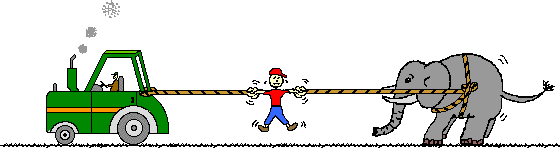# Lesson 4 : Newton's Third Law Of Motion

9 Questions | Total Attempts: 4933Settings• 1.
For every action, there is an equal and opposite reaction.
• A.

True

• B.

False

• 2.
According to Newton's third law, for every action force there is an equal (in size) and opposite (in direction) reaction force. Forces always come in pairs - known as "action-reaction force pairs."
• A.

True

• B.

False

• 3.
Identify at least six pairs of action-reaction force pairs in the following diagram.
• 4.
• 5.
Describe the other force in the action-reaction force pair.
• A.

The glove pushes the baseball rightward.

• B.

The glove pushes the baseball upward.

• C.

The glove pushes the baseball downward.

• D.

None of the above.

• 6.
Describe the other force in the action-reaction force pair.
• A.

Pin pushes bowling ball rightward.

• B.

Pin pushes bowling ball upward.

• C.

Pin pushes bowling ball downward.

• D.

None of the above.

• 7.
Describe the other force in the action-reaction force pair.
• A.

Balloon wall pushes enclosed air particles inwards.

• B.

Balloon wall pushes enclosed air particles outwards.

• C.

Balloon wall pushes enclosed air particles upwards.

• D.

None of the above.

• 8.
According to Newton's third law,The acceleration of an object as produced by a net force is directly proportional to the magnitude of the net force, in the same direction as the net force, and inversely proportional to the mass of the object.
• A.

True

• B.

False

• 9.
For every action, there is an equal and opposite reaction.
• A.

True

• B.

False

Related TopicsBack to top